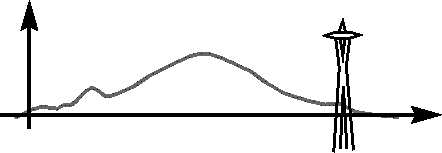WEST COAST OPTIMIZATION MEETING: SPRING 2016University of WashingtonHOME

SCHEDULE

ABSTRACTS

HOTELS

FOOD GUIDE

BANQUET

TRAVEL

MAPS

DEPARTMENT OF MATHEMATICS (UW)

UNIVERSITY OF WASHINGTON## Abstracts

• Damek Davis (Cornell)

Stochastic methods for nonsmooth nonconvex optimization

We prove that the proximal stochastic subgradient method, applied to a weakly convex problem (i.e. difference of convex function and a quadratic), drives the gradient of the Moreau envelope to zero at the rate $O(k^{-1/4})$. This class of problems captures a variety of nonsmooth nonconvex formulations, now widespread in data science. As a consequence, we obtain the long-sought convergence rate of the standard projected stochastic gradient method for minimizing a smooth nonconvex function on a closed convex set. In the talk, I will also highlight other stochastic methods for which we can establish similar guarantees.

• John Duchi (Stanford)

Epsilon epsilon

What is your favorite power of epsilon? As long as it is of the form $\epsilon^{-(k + 1) / k}$, I can give you matching upper and lower bounds in terms of the number of iterations of a $k$-th-order optimization method for finding an epsilon stationary point of a smooth but potentially non-convex function. In fact, in this talk, I probably will.

Based on joint work with Yair Carmon, Oliver Hinder, and Aaron Sidford

• Nicolas Flammarion (Berkeley)

Averaging Stochastic Gradient Descent on Riemannian Manifolds

We consider the minimization of a function defined on a Riemannian manifold $M$ accessible only through unbiased estimates of its gradients. We develop a geometric framework to transform a sequence of slowly converging iterates generated from stochastic gradient descent (SGD) on $M$ to an averaged iterate sequence with a robust and fast $O(\frac{1}{n})$ convergence rate. We then present an application of our framework to geodesically-strongly-convex (and possibly Euclidean non-convex) problems. Finally, we demonstrate how these ideas apply to the case of streaming $k$-PCA, where we show how to accelerate the slow rate of the randomized power method (without requiring knowledge of the eigengap) into a robust algorithm achieving the optimal rate of convergence.

• Zaid Harchaoui (U. Washington)

A Generic Quasi-Newton Acceleration Scheme for Convex Optimization

We propose a generic approach to accelerate gradient-based optimization algorithms with quasi-Newton principles. The proposed scheme, called QNing, can be applied to incremental first-order methods such as stochastic variance-reduced gradient (SVRG) or incremental surrogate optimization (MISO). It is also compatible with composite objectives, meaning that it has the ability to provide exactly sparse solutions when the objective involves a sparsity-inducing regularization. QNing relies on limited-memory BFGS rules, making it appropriate for solving high-dimensional optimization problems. Besides, it enjoys a worst-case linear convergence rate for strongly convex problems. We present experimental results where QNing gives significant improvements over competing methods for solving large-scale high-dimensional machine learning problems.

Joint work with Hongzhou Lin and Julien Mairal. Code available here github.com/hongzhoulin89/Catalyst-QNing.

• Zirui Zhou (SFU)

Algorithmic Development for Computing B-stationary Points of a Class of Nonsmooth DC Programs

In the first part of this talk, we study a convex-constrained nonsmooth DC program in which the concave summand of the objective is an infimum of possibly infinitely many smooth concave functions. We propose some algorithms by using nonmonotone linear search and extrapolation techniques for possible acceleration for this problem, and analyze their global convergence, sequence convergence and also iteration complexity. We also propose randomized counterparts for them and discuss their convergence.

In the second part we consider a class of DC constrained nonsmooth DC programs. We propose penalty and augmented Lagrangian methods for solving them and show that they converge to a B-stationary point under much weaker assumptions than those imposed in the literature.

This is joint work with Zhaosong Lu (SFU) and Zhe Sun (Jiangxi Normal University).

• Terry Rockafellar (U. Washington)

Progressive Decoupling of Linkages in Convex and Nonconvex Optimization

A method called the Progressive Decoupling Algorithm is described for solving optimization problems in which a subspace captures "linkages" that can be relaxed. The approach is inspired by the Progressive Hedging Algorithm in convex stochastic programming and resembles the Partial Inverse Method of Spingarn, but it retains more parametric flexibility than the latter. It is able even to work when convexity is not directly present but can be "elicited". The role of elicitation mimics that of "augmentation" in Lagrangian methods of multipliers. Applications can be made to problem decomposition and splitting.

• Yifan Sun (UBC)

Solving large low-rank SDPs through eigenspace pursuit

Solving semidefinite optimization problems (SDPs) in general is computationally challenging, but many fast methods exist when the true solution is known to be low rank. Ideally, if the eigenspace of the primal solution is already known, the problem reduces to a much smaller SDP. So, the problem of solving a large SDP with a low-rank solution can be reduced to finding this low-dimensional eigenspace. We offer a family of algorithms that searches for this eigenspace in the gauge dual formulation, using methods similar to those that greedily approximate basis pursuit for sparse vectors. We draw connections to methods used in low-rank matrix recovery via rank-1 updates in the primal space, offering primal and dual interpretations of this general class of “eigenspace pursuit” methods.

• Rekha Thomas (U. Washington)

Symmetric sums of squares over k-subset Hypercubes

Polynomial optimization over hypercubes has important applications in combinatorial optimization. We develop a symmetry-reduction method that finds sums of squares certificates for non-negative symmetric polynomials over k-subset hypercubes that improves on a technique due to Gatermann and Parrilo. For every symmetric polynomial that has a sos expression of a fixed degree, our method finds a succinct sos expression whose size depends only on the degree and not on the number of variables. Our results relate naturally to Razborov's flag algebra calculus for solving problems in extremal combinatorics. This leads to new results involving flags and their power in finding sos certificates in a finite setting, an angle that had not been explored before.

Joint work with: Annie Raymond (University of Massachusetts, Amherst) James Saunderson (Monash University, Australia) Mohit Singh (Georgia Institute of Technology, USA)